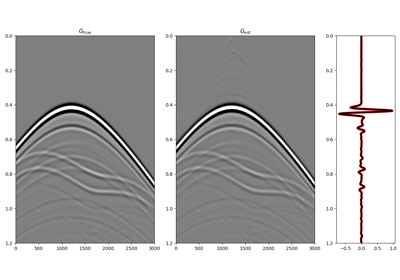# pylops.waveeqprocessing.Marchenko¶

class pylops.waveeqprocessing.Marchenko(R, R1=None, dt=0.004, nt=None, dr=1.0, nfmax=None, wav=None, toff=0.0, nsmooth=10, dtype='float64', saveRt=True, prescaled=False, fftengine='numpy')[source]

Marchenko redatuming

Solve multi-dimensional Marchenko redatuming problem using scipy.sparse.linalg.lsqr iterative solver.

Parameters: R : numpy.ndarray Multi-dimensional reflection response in time or frequency domain of size $$[n_s \times n_r \times n_t (n_{f_\text{max}})]$$. If provided in time, R should not be of complex type. Note that the reflection response should have already been multiplied by 2. R1 : bool, optional Deprecated, will be removed in v2.0.0. Simply kept for back-compatibility with previous implementation dt : float, optional Sampling of time integration axis nt : float, optional Number of samples in time (not required if R is in time) dr : float, optional Sampling of receiver integration axis nfmax : int, optional Index of max frequency to include in deconvolution process wav : numpy.ndarray, optional Wavelet to apply to direct arrival when created using trav toff : float, optional Time-offset to apply to traveltime nsmooth : int, optional Number of samples of smoothing operator to apply to window dtype : bool, optional Type of elements in input array. saveRt : bool, optional Save R and R.H to speed up the computation of adjoint of pylops.signalprocessing.Fredholm1 (True) or create R.H on-the-fly (False) Note that saveRt=True will be faster but double the amount of required memory prescaled : bool, optional Apply scaling to R (False) or not (False) when performing spatial and temporal summations within the pylops.waveeqprocessing.MDC operator. In case prescaled=True, the R is assumed to have been pre-scaled by the user. fftengine : str, optional New in version 1.17.0. Engine used for fft computation (numpy, scipy or fftw) TypeError If t is not numpy.ndarray.

MDC
Multi-dimensional convolution
MDD
Multi-dimensional deconvolution

Notes

Marchenko redatuming is a method that allows to produce correct subsurface-to-surface responses given the availability of a reflection data and a macro-velocity model .

The Marchenko equations can be written in a compact matrix form  and solved by means of iterative solvers such as LSQR:

$\begin{split}\begin{bmatrix} \Theta \mathbf{R} \mathbf{f_d^+} \\ \mathbf{0} \end{bmatrix} = \mathbf{I} - \begin{bmatrix} \mathbf{0} & \Theta \mathbf{R} \\ \Theta \mathbf{R^*} & \mathbf{0} \end{bmatrix} \begin{bmatrix} \mathbf{f^-} \\ \mathbf{f_m^+} \end{bmatrix}\end{split}$

Finally the subsurface Green’s functions can be obtained applying the following operator to the retrieved focusing functions

$\begin{split}\begin{bmatrix} -\mathbf{g^-} \\ \mathbf{g^{+ *}} \end{bmatrix} = \mathbf{I} - \begin{bmatrix} \mathbf{0} & \mathbf{R} \\ \mathbf{R^*} & \mathbf{0} \end{bmatrix} \begin{bmatrix} \mathbf{f^-} \\ \mathbf{f^+} \end{bmatrix}\end{split}$

Here $$\mathbf{R}$$ is the monopole-to-particle velocity seismic response (already multiplied by 2).

  Wapenaar, K., Thorbecke, J., Van der Neut, J., Broggini, F., Slob, E., and Snieder, R., “Marchenko imaging”, Geophysics, vol. 79, pp. WA39-WA57. 2014.
  van der Neut, J., Vasconcelos, I., and Wapenaar, K. “On Green’s function retrieval by iterative substitution of the coupled Marchenko equations”, Geophysical Journal International, vol. 203, pp. 792-813. 2015.
Attributes: ns : int Number of samples along source axis nr : int Number of samples along receiver axis shape : tuple Operator shape explicit : bool Operator contains a matrix that can be solved explicitly (True) or not (False)

Methods

 __init__(R[, R1, dt, nt, dr, nfmax, wav, …]) Initialize self. apply_multiplepoints(trav[, G0, nfft, rtm, …]) Marchenko redatuming for multiple points apply_onepoint(trav[, G0, nfft, rtm, …]) Marchenko redatuming for one point
apply_onepoint(trav, G0=None, nfft=None, rtm=False, greens=False, dottest=False, fast=None, usematmul=False, **kwargs_solver)[source]

Marchenko redatuming for one point

Solve the Marchenko redatuming inverse problem for a single point given its direct arrival traveltime curve (trav) and waveform (G0).

Parameters: trav : numpy.ndarray Traveltime of first arrival from subsurface point to surface receivers of size $$[n_r \times 1]$$ G0 : numpy.ndarray, optional Direct arrival in time domain of size $$[n_r \times n_t]$$ (if None, create arrival using trav) nfft : int, optional Number of samples in fft when creating the analytical direct wave rtm : bool, optional Compute and return rtm redatuming greens : bool, optional Compute and return Green’s functions dottest : bool, optional Apply dot-test fast : bool Deprecated, will be removed in v2.0.0 usematmul : bool, optional Use numpy.matmul (True) or for-loop with numpy.dot (False) in pylops.signalprocessing.Fredholm1 operator. Refer to Fredholm1 documentation for details. **kwargs_solver Arbitrary keyword arguments for chosen solver (scipy.sparse.linalg.lsqr and pylops.optimization.solver.cgls are used as default for numpy and cupy data, respectively) f1_inv_minus : numpy.ndarray Inverted upgoing focusing function of size $$[n_r \times n_t]$$ f1_inv_plus : numpy.ndarray Inverted downgoing focusing function of size $$[n_r \times n_t]$$ p0_minus : numpy.ndarray Single-scattering standard redatuming upgoing Green’s function of size $$[n_r \times n_t]$$ g_inv_minus : numpy.ndarray Inverted upgoing Green’s function of size $$[n_r \times n_t]$$ g_inv_plus : numpy.ndarray Inverted downgoing Green’s function of size $$[n_r \times n_t]$$
apply_multiplepoints(trav, G0=None, nfft=None, rtm=False, greens=False, dottest=False, usematmul=False, **kwargs_solver)[source]

Marchenko redatuming for multiple points

Solve the Marchenko redatuming inverse problem for multiple points given their direct arrival traveltime curves (trav) and waveforms (G0).

Parameters: trav : numpy.ndarray Traveltime of first arrival from subsurface points to surface receivers of size $$[n_r \times n_{vs}]$$ G0 : numpy.ndarray, optional Direct arrival in time domain of size $$[n_r \times n_{vs} \times n_t]$$ (if None, create arrival using trav) nfft : int, optional Number of samples in fft when creating the analytical direct wave rtm : bool, optional Compute and return rtm redatuming greens : bool, optional Compute and return Green’s functions dottest : bool, optional Apply dot-test usematmul : bool, optional Use numpy.matmul (True) or for-loop with numpy.dot (False) in pylops.signalprocessing.Fredholm1 operator. Refer to Fredholm1 documentation for details. **kwargs_solver Arbitrary keyword arguments for chosen solver (scipy.sparse.linalg.lsqr and pylops.optimization.solver.cgls are used as default for numpy and cupy data, respectively) f1_inv_minus : numpy.ndarray Inverted upgoing focusing function of size $$[n_r \times n_{vs} \times n_t]$$ f1_inv_plus : numpy.ndarray Inverted downgoing focusing functionof size $$[n_r \times n_{vs} \times n_t]$$ p0_minus : numpy.ndarray Single-scattering standard redatuming upgoing Green’s function of size $$[n_r \times n_{vs} \times n_t]$$ g_inv_minus : numpy.ndarray Inverted upgoing Green’s function of size $$[n_r \times n_{vs} \times n_t]$$ g_inv_plus : numpy.ndarray Inverted downgoing Green’s function of size $$[n_r \times n_{vs} \times n_t]$$

## Examples using pylops.waveeqprocessing.Marchenko¶10. Marchenko redatuming by inversion